# How do you represent dot and different types of three dots symbols in LaTeX?

You use dot symbols in different ways. Sometimes only dot symbols are used, sometimes more than one dot is used and dot symbols are used above and inside the letter.

In this tutorial, we will discuss in-depth the use of all kinds of dot symbols and latex commands.

## Simple dot symbol

In latex, the simple dot symbol is represented by the \cdot command. And you may have seen its use in vector dot products.

Properties Value
Symbol Dots
Package default
Argument No
Command \cdot
Example \cdot→•
\documentclass{article}
\begin{document}
$\cdot$
$\vec{\imath} \cdot \vec{\jmath}$
\end{document}

Output :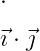Also, you can take the help of physics package. Where the \vdot command is used to define the vector dot product.

\documentclass{article}
\usepackage{physics}
\begin{document}
$\vdot$
$\vec{p} \vdot \vec{q}$
\end{document}

Output :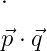## Latex three dots symbol

When you use n number elements to represent a mathematical expression. In that case, the three-dot symbol is used without marking each element in the expression.

Dots Symbol Command
Horizontal dots \ldots
Horizontal central dots \cdots
Vertical dots \vdots
Diagonal dots \ddots
Inverse Diagonal dots \iddots

Also, there are many commands. In this tutorial, we will only discuss the above five commands.

### 1. Horizontal dots on the line

You look at the program below. Three dots have been used along with the \ldots command.

\documentclass{article}
\begin{document}
$a_{1}, a_{2},...,a_{n}$
$a_{1}, a_{2},\ldots,a_{n}$
\end{document}

Output :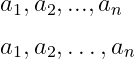In both cases, there is a difference between the output. In my opinion, it is better to use \ldots command instead of using three dots.

### 2. Horizontal dots above the line

For example, the \cdots command is used when the sum of n numbers is displayed.

\documentclass{article}
\begin{document}
$f(x)=x+ x^{2}+\cdots+x^{n}$
\end{document}

Output :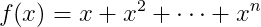I hope you understand the difference between \ldots and \cdots commands.

### 3. Vertical dots

Vertical dot consists of three-dot symbols, which are located vertically. And this symbol is represented by \vdots Command.

\documentclass{article}
\usepackage{amsmath}
\begin{document}
$\begin{bmatrix} a_{1}\\ a_{2}\\ \vdots\\ a_{n} \end{bmatrix}$
\end{document}

Output :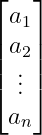You must install the amsmath package when denoting the matrix. And use the \usepackage command when installing a package.

### 4. Diagonal dots symbol

This symbol is located along the diagonal from N-W to S-E. And this symbol is mostly used to represent the elements located along the diagonal of the matrix.

\documentclass{article}
\usepackage{amsmath}
\begin{document}
\begin{bmatrix}
a_{1} &  & \\
& \ddots & \\
&  & a_{n}
\end{bmatrix}
\end{document}

Output :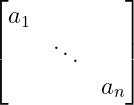If you look at the program above, you will understand that \ddots has been used for the diagonal dots symbol.

### 5. Inverse Diagnal dots symbol

The inverse diagonal dots symbol will be located along the S-W to N-E direction. There is more than one command to represent this symbol but this tutorial will only discuss \iddots commands.

\documentclass{article}
\usepackage{amsmath}
\usepackage{mathdots}
\begin{document}
$\begin{bmatrix} & & a_{1}\\ & \ddots & \\ a_{n} & & \end{bmatrix}$
\end{document}

Output :To use the \iddots command in Latex you need to install the mathdots package. Because there is no default command in latex to represent the inverse diagonal dots symbol.

## Latex dot derivative

When a variable is derivative in respect of time, the time derivative is represented by a dot symbol on the head of that variable.

\documentclass{article}
\begin{document}
$\frac{\mathit{d}r}{\mathit{d} t}=\dot{r}$
$\frac{\mathit{d}^{2}r}{\mathit{d} t^{2}}=\ddot{r}$
\end{document}


Output :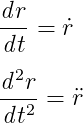#### Md Jidan Mondal

LaTeX expert with over 10 years of experience in document preparation and typesetting. Specializes in creating professional documents, reports, and presentations using LaTeX.